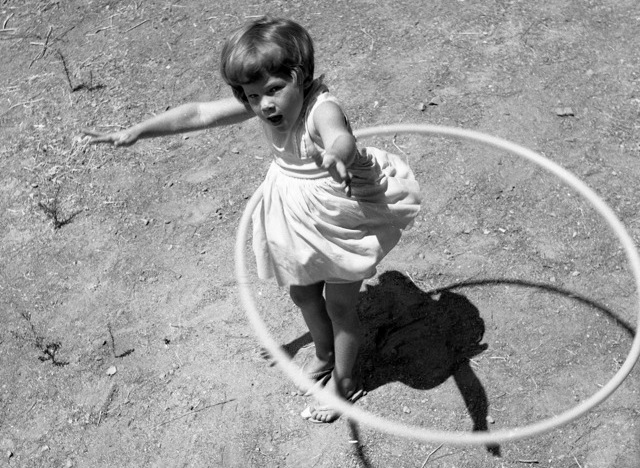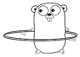# 5 basic for loop patterns

yourbasic.org/golang

A for statement is used to execute a block of code repeatedly.## Three-component loop

This version of the Go for loop works just as in C or Java.

``````sum := 0
for i := 1; i < 5; i++ {
sum += i
}
fmt.Println(sum) // 10 (1+2+3+4)``````
1. The init statement, `i := 1`, runs.
2. The condition, `i < 5`, is computed.
• If true, the loop body runs,
• otherwise the loop is done.
3. The post statement, `i++`, runs.
4. Back to step 2.

The scope of `i` is limited to the loop.

## While loop

If you skip the init and post statements, you get a while loop.

``````n := 1
for n < 5 {
n *= 2
}
fmt.Println(n) // 8 (1*2*2*2)``````
1. The condition, `n < 5`, is computed.
• If true, the loop body runs,
• otherwise the loop is done.
2. Back to step 1.

## Infinite loop

If you skip the condition as well, you get an infinite loop.

``````sum := 0
for {
sum++ // repeated forever
}
fmt.Println(sum) // never reached``````

## For-each range loop

Looping over elements in slices, arrays, maps, channels or strings is often better done with a range loop.

``````strings := []string{"hello", "world"}
for i, s := range strings {
fmt.Println(i, s)
}``````
``````0 hello
1 world``````

See 4 basic range loop patterns for a complete set of examples.

## Exit a loop

The break and continue keywords work just as they do in C and Java.

``````sum := 0
for i := 1; i < 5; i++ {
if i%2 != 0 { // skip odd numbers
continue
}
sum += i
}
fmt.Println(sum) // 6 (2+4)``````
• A continue statement begins the next iteration of the innermost for loop at its post statement (`i++`).
• A break statement leaves the innermost for, switch or select statement.